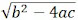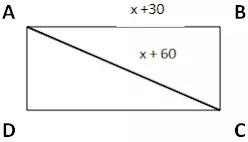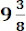# Class10 NCRT Quadratic Equations Exercise – 4.3 pdf || UP Board

1. Find the roots of the following quadratic equations, if they exist, by the method of completing the square:

(i) 2x2 – 7x + 3 = 0

dividing by 2,

x2 – 7/2 x + 3/2 = 0

x2 – 7/2 x = - 3/2

[a2 + b2 – 2ab = (a + b)2]

Either, x – 7/4 = 5/4, x – 7/4 = -5/4

x = 7/4 + 5/4, x = 7/4 – 5/4

x = 12/4 = 3, x = 2/4 = 1/2

Hence, the roots of quadratic equations are 3 and 1/2.

(ii) 2x2 + x - 4 = 0

dividing by 2,

x2 + 1/2 x - 2 = 0

x2 + 1/2 x = 2

Either, x + ¼ = √33/4, x + ¼ = -√33/4

x = (√33 – 1)/4, x = (-√33 – 1)/4

Hence, the roots of the quadratic equations are (√33 1)/4 and (-√33 1)/4.

(iii) 4x2 + 4√3 x + 3 = 0

dividing by 4,

x2 + √3 x + 3/4 = 0

x2 + √3 x = - 3/4

Either,  x = -√3/2

Hence, the roots of the quadratic equations are -√3/2 and -√3/2.

(iv) 2x2 + x + 4 = 0

dividing by 2,

x2 + 1/2 x + 4 = 0

x2 + 1/2 x = - 2

But, we know that (x + 1/4)2 cannot be negative for any real value of x, so no real value of x can satisfy the given equation. Hence the root of the given equation does not exist.

2. Find the roots of the quadratic equations gives in Q. 1 above by applying the quadratic formula.

(i) 2x2 – 7x + 3 =0

2x2 – 7x + 3 =0

Comparing with the general quadratic equation ax2 + bx + c = 0,

Here, a = 2, b = -7, c = 3

Therefore, b2 – 4ac = (-7)2 – 4 × 2 × 3 = 49 - 24 = 25 > 0

Or x = (7 + 5)/4, x = (7 – 5)/4

x = 12/4, x = 2/4

x = 3, x = ½

Hence, the roots of quadratic equations are 3 and ½.

(ii) 2x2 + x – 4 = 0

2x2 + x – 4 = 0, Here a = 2, b = 1, c = -4

Therfore, b2 – 4ac = (1)2 – 4 × 2 × -4 = 1 + 32 = 33 > 0

Hence, the roots of the quadratic equations are (√33 1)/4 and (-√33 1)/4.

(iii) 4x2 + 4√3 x + 3 = 0

4x2 + 4√3 x + 3 = 0, Here a = 4, b = 4√3, c = 3

Therefore b2 – 4ac = (4√3)2 – 4 × 4 × 3 = 48 - 48 = 0

Or x = -(4√3)/8

x = -√3/2

Hence, the roots of the quadratic equations are -√3/2 and -√3/2.

(iv) 2x2 + x + 4 = 0

2x2 + x + 4 = 0, Here a = 2, b = 1, c = 4

Therefore, b2 – 4ac = (1)2 – 4 × 2 × 4 = 1 - 32 = -31 < 0

However, the square of a real number cannot be negative, so the value ofis not real.

Hence, the root of the given equation does not exist.

3. Find the roots of the following equations:

(i) x – 1/x = 3, x ≠ 0

x2 – 1 = 3x

x2 – 3x – 1 = 0

x2 – 3x – 1 = 0, Here a = 1, b = -3, c = -1

Therefore, b2 – 4ac = (-3)2 – 4 × 1 × -1 = 9 + 4 = 13 > 0

Or x = (3 + √13)/2, x = (3 - √13)/2

Hence, the roots of the quadratic equations are (3 + √13)/2 and (3 - √13)/2.

x2 – 3x – 28 = -30

x2 – 3x + 2 = 0

x2 – 3x + 2 = 0, Here a = 1, b = -3, c = 2

Therefore, b2 – 4ac = (-3)2 – 4 × 1 × 2 = 9 - 8 = 1 > 0

Or x = (3 + 1)/2, x = (3 – 1)/2

x = 4/2, x = 2/2

x = 2, x = 1

Hence, the roots of quadratic equations are 2 and 1.

4. The sum of the reciprocals of Rehman’s ages, (in years) 3 years ago and 5 years from now is 1/3. Find his present age.

Let Rehman's present age = x years

Hence, age 3 years ago = x – 3 years

Hence, age after 5 years = x + 5 years

According to Question,

x2 + 2x – 15 = 6x +6

x2 – 4x – 21 = 0

x2 – 4x – 21 = 0, Here a = 1, b = -4, c = -21

Therefore, b2 – 4ac = (-4)2 – 4 × 1 × -21 = 16 + 84 = 100 > 0

Or, x = (4 + 10)/2, x = (4 – 10)/2

x = 14/2, x = -6/2

x = 7, x = -3

Since age cannot be negative, Rehman's present age is 7 years.

5. In a class test, the sum of Shefali’s marks in Mathematics and English is 30. Had she got 2 marks more in Mathematics and 3 marks less in English, the product of their marks would have been 210. Find her marks in the two subjects.

Let Shefali's marks in Mathematics = x

Hence, Shefali's marks in English = 30 – x

If he had got 2 marks more in Mathematics and 3 marks less in English, then

Marks in Mathematics = x + 2

Marks in English = 30 – x – 3

According to Question,

Product = (x + 2)(27 – x)

210 = (x + 2)(27 – x)

27x – x2 + 54 – 2x = 210

– x2 – 25x – 156 = 0

x2 – 25x + 156 = 0

x2 – 12x – 13x + 156 = 0

x(x – 12) – 13(x – 12) = 0

(x – 12)(x – 13) = 0

Or, x – 12 = 0, x – 13 = 0

x = 12, x = 13

If x = 12, then marks in maths = 12, marks in English = 3012 = 18

If x = 13, then marks in maths = 13, marks in English = 30 3 = 17

6. The diagonal of a rectangular field is 60 metres more than the shorter side. If the longer side is 30 metres more than the shorter side, find the sides of the field.

Let short side = x m

Hence, diagonal = x + 60 m

and longer side = x + 30 mAccording to Question,

(x + 60)2 = x2 + (x + 30)2

x2 + 120x + 3600 = x2 + x2 + 60x + 900

-x2 + 60x + 2700 = 0

x2 – 60x – 2700 = 0

x2 – 90x + 30x – 2700 = 0

x(x – 90) + 30(x – 90) = 0

(x – 90)(x + 30) = 0

x – 90 = 0, x + 30 = 0

x = 90, x = -30

But x ≠ -30, since x is the side of the field and will not be negative.

So, x = 90

So, shorter side = 90 m

and longer side = 90 + 30 = 120 m

7. The difference of squares of two numbers is 180. The square of the smaller number is 8 times the larger number. Find the two numbers.

Let the smaller number = y

and larger number = x

So,

y2 = 8x

According to Question,

x2 – y2 = 180

x2 – 8x = 180                [y2 = 8x]

x2 – 8x – 180 = 0

x2 – 18x + 10x -180 = 0

x(x – 18) + 10(x – 18) = 0

(x – 18)(x + 10) = 0

x – 18 = 0, x + 10 = 0

x = 18, x = -10

But x ≠ -10, because x is a large number and will not be negative.

So, x = 18

So, smaller side = y ⇒ y2 = √8x

⇒ y = √8x

⇒ y = √8×18

⇒ y = √144

⇒ y = 12

And larger side = 18

8. A train travels 360 km at a uniform speed. If the speed had been 5 km/h more, it would have taken 1 hour less for the same journey. Find the speed of the train.

Let speed of train = x km/h

and distance covered = 360 km

so time t1 = 360/x hours  [ time = distance / speed ]

If the speed had been 5 km/h more, then the time t2 = 360/(x + 5) hours

According to Question,

360x + 1800 – 360x = x(x + 5)

1800 = x2 + 5x

x2 + 5x – 1800 = 0

x2 + 45x – 40x – 1800 = 0

x(x + 45) – 40(x + 45) = 0

(x + 45)(x – 40) = 0

x + 45 = 0, x – 40 = 0

x = -45, x = 40

But x ≠ -45, because x is the speed of the train and will not be negative.

Therefore, x = 40

Hence, the speed of the train is 40 km/h.

9. Two water taps together can fill a tank inhours. The tap of larger diameter takes 10 hours less than the smaller one to fill the tank separately. Find the time in which each tap can separately fill the tank.

Let the time taken by the larger diameter tap = x hours

and time taken by the tap of smaller diameter = x + 10 hours

Therefore, the tank filled by the larger diameter tap in 1 hour = 1/x

And the tank filled by the smaller diameter tap in 1 hour = 1/(x + 10)

According to Question,

75(2x + 10) = 8x(x + 10)

150x + 750 = 8x2 + 80x

8x2 – 70x – 750 = 0

4x2 – 35x – 375 = 0

4x2 – 60x + 25x – 375 = 0

4x(x – 60) + 25(x – 15) = 0

(x – 15)(x + 25) = 0

x – 15 = 0, x + 25 = 0

x = 15, x = -25

But x ≠ -25, because x is the time to fill the tank and it will not be negative.

Therefore, x = 15

Hence, time taken by the tap of larger diameter = 15 hours

And time taken by smaller diameter tap = 15 + 10 hours

10. An express train takes 1 hour less than a passenger train to travel 132 km between Mysore and Bangalore (without taking into consideration the time they stop at intermediate stations). If the average speed of the express train is 11 Km/h more than that of the passenger train, find the average speed of the two trains.

Let the average speed of the passenger train = x km/h

Hence, average speed of express train = x + 11 km/h

Distance covered = 132 km

Hence, the time taken by the passenger train t1 = 132/x hours  [ time = distance / speed ]

and the time taken by the express train t2 = 132/(x + 11) hours

According to Question,

132x + 1452 – 132x = x(x + 11)

1452 = x2 + 11x

x2 + 11x – 1452 = 0

x2 + 44x – 33x – 1452 = 0

x(x + 44) – 33(x + 44) = 0

(x + 44)(x – 33) = 0

x + 44 = 0, x – 33 = 0

x = - 44, x = 33

But x ≠ -44, since x is the speed of the train and will not be negative.

Therefore, x = 33

Hence, average speed of passenger train = 33 km/h

And, average speed of express train = 33 + 11 = 44 km/h

11. Sum of the areas of two squares is 468 m2. If the difference of their perimeters is 24 m, find the sides of the two squares.

Let the side of the larger square = x m

Let the side of the smaller square = y m

According to Question,

x2 + y2 = 468 ……………(i)

difference of dimensions,

4x – 4y = 24

x – y = 6

x = 6 + y    ………………(ii)

Substituting the value of x in equation (i),

(y + 6)2 + y2 = 468

y2 + 12y + 36 + y2 = 468

2y2 + 12y – 432 = 0

y2 + 6y – 216 = 0

y2 +18y – 12y – 216 = 0

y(y + 18) – 12 (y + 18) = 0

(y + 18)(y – 12) = 0

y + 18 = 0, y – 12 =0

y = -18, y = 12

But x ≠ -18, since x is the side of the square and will not be negative.

Therefore, x = 12

Hence, side of smaller square = 12 m

Substituting the value of y in equation (ii),

Side of the larger square = x = y + 6 = 12 + 6 = 18 m